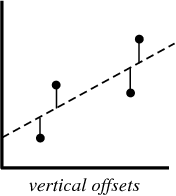# Introduction to Linear Regression

Hey - Nick here! This page is a free excerpt from my new eBook Pragmatic Machine Learning, which teaches you real-world machine learning techniques by guiding you through 9 projects.

Linear regression is the first type of machine learning model that we will build in the this course.

This tutorial serves as a brief introduction to the theory behind linear regression in Python. We'll learn how to code a Python linear regression algorithm later in this course.

## The History of Linear Regression

Linear regression was created in the 1800s by Francis Galton.

Galton was a scientist studying the relationship between parents and children. More specifically, Galton was investigating the relationship between the heights of fathers and the heights of their sons.

Galton's first discovery was that sons tended to be roughly as tall as their fathers. This is not surprising.

Later on, Galton discovered something much more interesting. The son's height tended to be closer to the overall average height of all people.

Galton gave this phenomenon a name: regression. Specifically, he said "A father's son's height tends to regress (or drift towards) the mean (average) height".

This led to an entire field in statistics called regression. We will learn about the fundamental underpinnings of regression in the next section of this lesson.

## The Mathematics of Linear Regression

When creating a regression model, all that we are trying to do is draw a line that is closest to each point in a data set.

The typical example of this is the "least squares method" of linear regression, which only calculates the closeness of a line in the up-and-down direction.

Here is an example to help illustrate this:When you create a regression model, your end product is an equation that you can use to predict the y-value of an x-value, without actually knowing the y-value in advance.

## Final Thoughts

This tutorial provided you with your first introduction to linear regression in Python. While this lesson was brief and did not actually contain any code, we'll dig deeper by learning how to code linear regression models in the next section of this course.